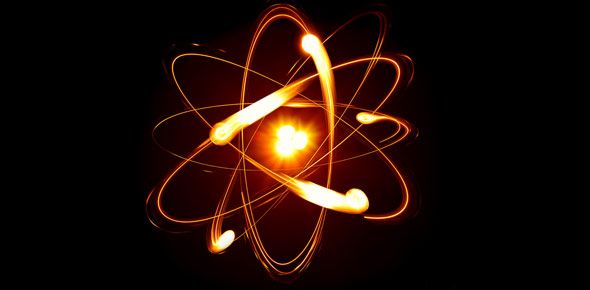# Thermodynamics - Problems In First Law Of Thermodynamics

10 Questions | Total Attempts: 447Settings• 1.
A pump discharges a liquid into a drum at the rate of 0.032 m3/s. The drum, 1.50 m in diameter and 4.20 m in length, can hold 3000 kg of the liquid. Find the mass flow rate of the liquid handled by the pump.
• A.

12.945 kg/s

• B.

10.945 kg/s

• C.

129.45 kg/s

• D.

1294.5 kg/s

• 2.
The piston of an oil engine, of area 0.0045 m2, moves downwards 75 mm, drawing in 0.00028 m3 of fresh air from the atmosphere. The pressure in the cylinder is uniform during the process at 80 kPa, while the atmospheric pressure is 101.325 kPa, the difference being due to the flow resistance in the induction pipe and the inlet valve. Estimate the displacement work done by the air finally in the cylinder.
• A.

270 J

• B.

27 J

• C.

300 J

• D.

30 J

• 3.
An engine cylinder has a piston of area 0.12 m3 and contains gas at a pressure of 1.5 MPa. The gas expands according to a process which is represented by a straight line on a pressure-volume diagram. The final pressure is 0.15 MPa. Calculate the work done by the gas on the piston if the stroke is 0.30 m.
• A.

29.7 J

• B.

27 J

• C.

300 J

• D.

30 J

• 4.
The flow energy of 0.124 m3/min of a fluid crossing a boundary to a system is 18 kW. Find the pressure at this point.
• A.

8.71 Mpa

• B.

871 Pa

• C.

10.71 Mpa

• D.

30 Mpa

• 5.
A milk chilling unit can remove heat from the milk at the rate of 41.87 MJ/h. Heat leaks into the milk from the surroundings at an average rate of 4.187 MJ/h. Find the time required for cooling a batch of 500 kg of milk from 45°C to 5°C. Take the cp of milk to be 4.187 kJ/kg K.
• A.

3 hr. 23 min. 20 sec.

• B.

4 hr. 13 min. 20 sec.

• C.

2 hr. 13 min. 20 sec.

• D.

2 hr

• 6.
680 kg of fish at 5°C are to be frozen and stored at – 12°C. The specific heat of fish above freezing point is 3.182, and below freezing point is 1.717 kJ/kg K. The freezing point is – 2°C, and the latent heat of fusion is 234.5 kJ/kg. what per cent of this is latent heat?
• A.

70%

• B.

70%

• C.

85.60%

• D.

70%

• 7.
In a cyclic process, heat transfers are + 14.7 kJ, – 25.2 kJ, – 3.56 kJ and + 31.5 kJ. What is the net work for this cyclic process?
• A.

18 KJ

• B.

1744 J

• C.

17.44 KJ

• D.

174.4 KJ

• 8.
During one cycle the working fluid in an engine engages in two work interactions: 15 kJ to the fluid and 44 kJ from the fluid, and three heat interactions, two of which are known: 75 kJ to the fluid and 40 kJ from the fluid. Evaluate the magnitude and direction of the third heat transfer.
• A.

6kJ from the system

• B.

6kJ to the system

• C.

12kJ from the system

• D.

12kJ to the system

• 9.
A domestic refrigerator is loaded with food and the door closed. During a certain period the machine consumes 1 kWh of energy and the internal energy of the system drops by 5000 kJ. Find the net heat transfer for the system.
• A.

6kJ from the system

• B.

8.6MJ to the system

• C.

8.6 kJ from the system

• D.

12kJ to the system

• 10.
1.5 kg of liquid having a constant specific heat of 2.5 kJ/kg K is stirred in a well-insulated chamber causing the temperature to rise by 15°C. Find W for the process.
• A.

6kJ from the system

• B.

8.6MJ to the system

• C.

56. 25 KJ from the system

• D.

56. 25 KJ to the system

Related TopicsBack to top